# Electrostatics : Electric Charges and Fields Class 12 Important Questions

In this page we have Electrostatics Important Questions for Class 12 physics . Answers to most of the questions are given. Try to first solve them without looking at answers. THis would be good for practicing questions for your board exams. This electric charge and electric field questions and answers page contains different types of questions that possibly come in exams.

## Important Questions for Class 12 Physics Chapter 1 Electric Charges and Fields Class 12 Important Questions

Question 1
Derive an expression for the electric field at a point
a. the axial position of an electric dipole.
b. on the equatorial position of an electric dipole.

Derive an expression for the torque on an electric dipole in a uniform electric field.

Question 2
State Gauss theorem and apply it to find the electric field due to a uniformly charged spherical conducting shell at a point
a. Outside the shell
b. Inside the shell
c. on the shell
Also Draw a graph showing a variation of electric field E with distance r from center of the uniformly charged spherical conducting shell

Question 3
State Gauss theorem and apply it to find the electric field intensity due to a infinitely long straight wire of linear charge charge density $\lambda$ C/m

Question 4
State Coulomb's law and express it in vector form. Derive it using Gauss theorem.

Question 5
A charge q is uniformly distributed over a ring of radius r. Derive an expression for electric field at a point on the axis on the ring. Also shows that for point at large distance from center of the ring,it behaves like a point charge only

## Objective Questions on electric charges and fields (MCQs)

Question 6
Five charges $q_1$ , $q_2$ , $q_3$ , $q_4$, and $q_5$ are fixed at their positions as shown in below figure. S is a Gaussian surface. The Gauss's law is given by
$\oint \mathbf{E}.d\mathbf{s}=\frac {q}{\epsilon_0}$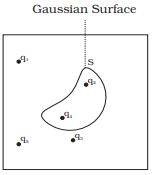Which of the following statements is correct?
(a) E on the LHS of the above equation will have a contribution from $q_1$, $q_5$ and $q_3$ while q on the RHS will have a contribution from $q_2$ and $q_4$ only.
(b) E on the LHS of the above equation will have a contribution from all charges while q on the RHS will have a contribution from $q_2$ and $q_4$ only.
(c) E on the LHS of the above equation will have a contribution from all charges while q on the RHS will have a contribution from $q_1$, $q_3$ and $q_5$ only.
(d) Both E on the LHS and q on the RHS will have contributions from $q_2$ and $q_4$ only

Question 7
The electric field intensity at a point situated 4 meters from a point charge is 100 N/C. If the distance is reduced to 1 meters, the field intensity will be
(a) 400 N/C
(b) 600 N/C
(c) 800 N/C
(d) 1600 N/C

Question 8
Three positive charges of equal value q are placed at the vertices of an equilateral triangle. The resulting lines of force should be sketched as in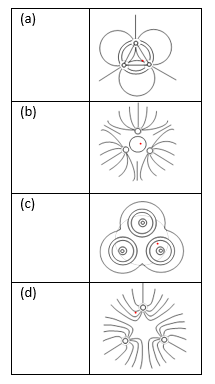Question 9
The force between two charges is 120 N. If the distance between the charges is doubled, the force will be
(a) 60 N
(b) 30 N
(c) 40 N
(d) 15 N

Question 10
The lines of force due to charged particles are
(a) always straight
(b) always curved
(c) sometimes curved
(d) none of the above

Question 11
An arbitrary surface encloses a dipole. What is the electric flux through this surface?
a. Zero
b. $\frac {2q}{\epsilon_0}$
c. $\frac {q}{\epsilon_0}$
d. Cannot say with the given data

Question 12
SI unit of electrical permittivity of Space
(a) $C^2/Nm^2$
(b) $C/Nm^2$
(c) $C^2/Nm$
(d) $C^2/N^2m$

Question 13
Dimensional Formula for electrical permittivity of Space
(a) $[M^{-1}L^{-3}T^{4}A^2]$
(b)$[M^{-1}L^{-3}T^{4}A^1]$
(c)$[M^{-1}L^{-2}T^{2}A^2]$
(d) $[M^{-1}L^{-3}T^{2}A^2]$

Question 14
Which is of these is not vector quantity
a. Electric Field
b. Electric Dipole Moment
c. Electric Flux
d. Force

Question 15
Dimensional Formula for electric Field
(a) $[M^{-1}L^{-3}T^{4}A^2]$
(b)$[M^{-1}L^{-3}T^{4}A^1]$
(c)$[M^{-1}L^{-2}T^{2}A^2]$
(d) $[M^{1}L^{1}T^{-3}A^{-1}]$

Question 16
Two point charges placed at a distance R in air exert a force F on each other. At what distance will these experience the same force F in a medium of dielectric constant K?
(a) $\frac {R}{K}$
(b) $\frac {R}{K^2}$
(c) $\frac {R}{\sqrt {K}}$
(d) $R \sqrt {K}$

Question 17
Let F is the force between two equal point charges at some distance. If the distance between them is doubled and individual charges are also doubled, what will be force acting between the charges?
(a) F
(b) 2F
(c) 4F
(d) F/2

Question 18
If $\oint \mathbf{E}.d\mathbf{s}=0$ over a surface, then
(a) the electric field inside the surface and on it is zero.
(b) the electric field inside the surface is necessarily uniform.
(c) the number of flux lines entering the surface must be equal to the number of flux lines leaving it.
(d) all charges must necessarily be outside the surface.

Question 19
The electric field due to a uniformly charged sphere of radius R as a function of the distance from its centre is represented graphically by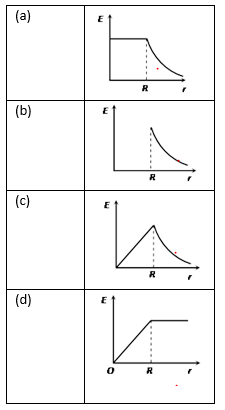Question 20
Which of the following graphs shows the variation of electric field E due to a hollow spherical conductor of radius R as a function of distance from the centre of the sphere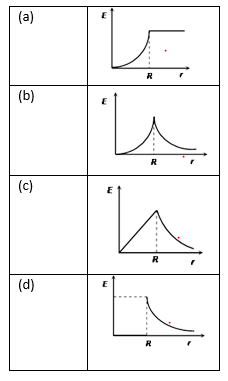>

## Numerical Questions

Question 21
A charge q is placed at the center of the line joining two equal charges Q. Show that that system of three charges will be in equilibrium if 4q + Q=0

Question 22
A sphere $A_1$ of radius $R_1$ encloses a charge 2Q. If there is another concentric sphere $A_2$ of radius $R_2$ ($R_2 > R_1$) and there will be no additional charges between $A_1$ and $A_2$. Find the ratio of the electric Flux through $A_1$ and $A_2$

Question 23
Given a uniformly charged plane/ sheet of surface charge density $\sigma = 2 \times 10^{17}$ C/m2
(i) Find the electric field intensity at a point A, 5mm away from the sheet on the left side.
(ii) Given a straight line with three points X, Y & Z placed 50 cm away from the charged sheet on the right side. At which of these points, the field due to the sheet remain the same as that of point A and why?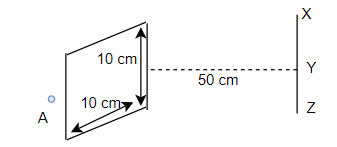Question 24
A point charge +2Q is placed at the centre O of an uncharged hollow spherical conductor of inner radius $p$ and outer radius $q$. Find the following:
(a) The magnitude and sign of the charge induced on the inner and outer surface of the conducting shell.
(b) The magnitude of electric field vector at a distance (i) r =p/2 and (ii) r = 2q, from the centre of the shell.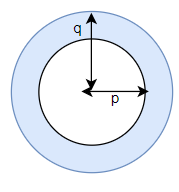Question 25
Two charges $\pm 10 \mu C$ are placed 5.00 mm apart. Determine the electric field at
(a) A point P on this axis of the dipole 15 cm away from its center O on the side of the positive charge
(b) A point Q , 15 cm away from O on a line passing through O and normal to the axis of the dipole

Question 26
A spherical liquid drop of radius r has charge q. If n number of such drops coalesces to form a single bigger drop , then on the surface of bigger drop what is
(1) charge
(2) charge density
(3) electric field
(4) potential

Question 27
Five Charges of equal amount (q) are placed at five corners of a regular hexagon of side 20 cm. What will be the value of sixth charge placed at sixth corner of the hexagon so that the electric field at the center of hexagon is zero ?.

Question 28
An electric charge q is placed at one of the corner of a cube of side $a$. What will be the electric flux through its one of the face?

Question 29
A charge Q is divided in two parts q and Q - q separated by a distance R. If force between the two charges is maximum, find the relationship between q & Q.

Question 30
A thin stationary ring of 1 m has a positive charge of $1 \times 10^{-5}$ C uniformly distributed over it. A particle of mass 0.9 gm and having a negative charge of $1 \times 10^{-6}$ C is placed on the axis at a distance 1 cm from the center of the ring. Show that the motion of the negatively charged particle is approximately simple harmonic. Calculate the time period of oscillation.

Question 31
A certain region has spherical symmetry of electric field. Name the charge distribution producing such a field.

Question 32
Represent graphically the variation of electric field with distance, for a uniformly charged sphere.

Question 33
How will the radius of a flexible ring change if it is given positive charge?